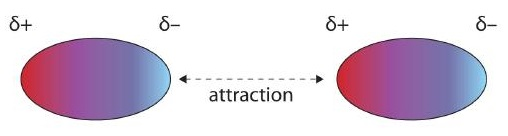14.2: Intermolecular Forces

Learning Objective

• Relate phase to intermolecular forces.

Why does a substance have the phase it does? The preferred phase of a substance at a given set of conditions is a balance between the energy of the particles and intermolecular forces (or intermolecular interactions) between the particles. If the forces between particles are strong enough, the substance is a liquid or, if stronger, a solid. If the forces between particles are weak and sufficient energy is present, the particles separate from each other, so the gas phase is the preferred phase. The energy of the particles is mostly determined by temperature, so temperature is the main variable that determines what phase is stable at any given point.

What forces define intermolecular interactions? There are several. A force present in all substances with electrons is the dispersion force (sometimes called the London dispersion force, after the physicist Fritz London, who first described this force in the early 1900s). This interaction is caused by the instantaneous position of an electron in a molecule, which temporarily makes that point of the molecule negatively charged and the rest of the molecule positively charged. In an instant, the electron is now somewhere else, but the fleeting imbalance of electric charge in the molecule allows molecules to interact with each other. As you might expect, the greater the number of electrons in a species, the stronger the dispersion force; this partially explains why smaller molecules are gases and larger molecules are liquids and solids at the same temperature. (Mass is a factor as well.)

Molecules with a permanent dipole moment experience dipole-dipole interactions, which are generally stronger than dispersion forces if all other things are equal. The oppositely charged ends of a polar molecule, which have partial charges on them, attract each other (Figure $$\PageIndex{1}$$). Thus a polar molecule such CH2Cl2 has a significantly higher boiling point (313 K, or 40°C) than a nonpolar molecule like CF4 (145 K, or −128°C), even though it has a lower molar mass (85 g/mol vs. 88 g/mol).Figure $$\PageIndex{1}$$: Dipole-Dipole Interactions. Oppositely charged ends of polar molecules attract each other.

An unusually strong form of dipole-dipole interaction is called hydrogen bonding. Hydrogen bonding is found in molecules with an H atom bonded to an N atom, an O atom, or an F atom. Such covalent bonds are very polar, and the dipole-dipole interaction between these bonds in two or more molecules is strong enough to create a new category of intermolecular force. Hydrogen bonding is the reason water has unusual properties. For such a small molecule (its molar mass is only 18 g/mol), H2O has relatively high melting and boiling points. Its boiling point is 373 K (100°C), while the boiling point of a similar molecule, H2S, is 233 K (−60°C). This is because H2O molecules experience hydrogen bonding, while H2S molecules do not. This strong attraction between H2O molecules requires additional energy to separate the molecules in the condensed phase, so its boiling point is higher than would be expected. Hydrogen bonding is also responsible for water's ability as a solvent, its high heat capacity, and its ability to expand when freezing; the molecules line up in such a way that there is extra space between the molecules, increasing its volume in the solid state (Figure $$\PageIndex{2}$$).Figure $$\PageIndex{2}$$: Hydrogen Bonding. When water solidifies, hydrogen bonding between the molecules forces the molecules to line up in a way that creates empty space between the molecules, increasing the overall volume of the solid. This is why ice is less dense than liquid water.

Example $$\PageIndex{1}$$:

Identify the most significant intermolecular force in each substance.

1. C3H8
2. CH3OH
3. H2S

Solution

1. Although C–H bonds are polar, they are only minimally polar. The most significant intermolecular force for this substance would be dispersion forces.
2. This molecule has an H atom bonded to an O atom, so it will experience hydrogen bonding.
3. Although this molecule does not experience hydrogen bonding, the Lewis electron dot diagram and VSEPR indicate that it is bent, so it has a permanent dipole. The most significant force in this substance is dipole-dipole interaction.

Exercise $$\PageIndex{1}$$

Identify the most significant intermolecular force in each substance.

1. HF
2. HCl